Warning

## Taught competencies and skills

By attending the lab and solving the assignment, a student should…

• know the formal definition of the minimax task.
• understand the relation between the likelihood ratio and the sought strategy.
• be able to solve simple discrete and continuous two-class problems.
• be aware of the relation between the minimax task and the Bayesian strategies.

The Bayesian formulation has some limits discussed at the lecture and for certain situations one may need to formulate the problem e.g. as a Neyman-Pearson task, Wald task or a Minimax task. Out of this spectrum of problem formulations we will focus on the Minimax task here. You should be able to apply parts of the proposed solution to the other problem formulations on your own.

We will continue with our simple OCR motivation from the previous assignment. Lets consider a situation when we are trying to recognise licence plates. For simplicity we again stick to the simple two-letter alphabet and 10×10 already segmented letters. The situation in the Bayes task then corresponds to a problem of designing a recognition system for a particular crossroad where the a priori probability of the two letters is known or could be measured.

In today's assignment we aim at designing a recognition system which could be deployed anywhere in the Czech republic. The licence plates in different cities use different combination of the letters, so the a priori probabilities differ as well. Obviously, it is not practical to find the optimal Bayesian strategy for every camera position.

As you might have already guessed, the situation is ideal for the Minimax strategy. Here, the a priori probability exists, but is not known.

We will consider two cases: (a) discrete measurements, and (b) continuous measurements. For the first case we follow the likelihood ratio based search for the optimal strategy as suggested in the lecture. For the continuous case, the solution is usually found analytically or numerically. Instead, we deliberately take a small detour and show some interesting relations to the Bayes task while deriving the optimal strategy.

To fulfil this assignment, you need to submit these files (all packed in one .zip file) into the upload system:

• minimax.ipynb - a notebook for data initialisation, calling of the implemented functions and plotting of their results (for your convenience, will not be checked).
• minimax.py - file with the following methods implemented:
• minimax_strategy_discrete - function which finds the optimal Minimax strategy for discrete measurements
• classify_discrete - function for using the discrete strategy for image classification
• risk_fix_q_cont - function for computing risk for a fixed strategy but changing a priori probability
• worst_risk_cont - worst case risk for a Bayesian strategy trained with a particular a priori probability but evaluated on all a priori probabilities
• minmax_strategy_cont - function which finds the optimal Minimax strategy for continuous measurements
• all functions from the previous assignment (bayes) - Unfortunately, including bayes.py and import from it is not supported. You have to copy your functions from bayes.py to minimax.py.
• minimax_lr_search_discrete.png, minimax_strategy_discrete.png, minimax_classif_discrete.png, plots_cont.png and minimax_classif_cont.png - images specified in the tasks

Use template of the assignment. When preparing a zip file for the upload system, do not include any directories, the files have to be in the zip file root. Do not forget to git pull before starting the work.

It is not possible to import your previously implemented functions from bayes.py in BRUTE, please copy all the necessary functions directly into minimax.py. Sorry for the inconvenience.

## Discrete measurements

In this section we will be using $x\in X$ and $y\in Y$ measurements computed by compute_measurement_lr_discrete and compute_measurement_ul_discrete respectively. Each of them produces integer values in the range $[-10, 10]$.

The probability functions are stored in the discrete variable. For instance for letter “A”, discrete['A'] contains $p_{X,Y|k}(x,y|A)$ given as a (21, 21) numpy array (the size corresponds to the cardinality of $X \times Y$).

We will select only two letters from the alphabet and consider only the two-class problem here.

Recall from the lecture slides, that the Minimax task could be solved by finding a strategy corresponding to a single threshold $\mu$ on the likelihood ratio (LR) $r = \frac{p(x, y|1)}{p(x, y|2)}$. Since the measurements are discrete and their domain finite, we have only a limited number of LR values $r_i$, one for every $x,y$ combination. Let us assume that the indexing $i$ of discrete features $(x,y)$ corresponds to likelihood ratios sorted by ascent: $r_i \leq r_{i+1}$ (we can indeed start from any indexing of discrete pairs $(x,y)$, then apply the sorting to get the sorting permutation). Then any strategy based on thresholding of the likelihood ratio can be represented in the form \begin{align}\label{q1} q(i) = \begin{cases} 1\ \ & \text{if}\ \ \ i>i^*,\\ 2\ \ & \text{if}\ \ \ i\leq i^*; \end{cases} \end{align} for some $i^* \in [-1,N)$, where $N=|X||Y|$.

In the Minimax task we are looking for an index $i^*$, for which $\max(\epsilon_1(i), \epsilon_2(i))$ is minimal. The values $\epsilon_1$ and $\epsilon_2$ are defined by equation (28) in the lecture slides, where the strategy $q$ in the form \eqref{q1} is substituted.

This representation slightly differs from the lecture slides in that even for likelihood ratios that are the same, i.e. $r_i = r_{i+1}$, there still exist distinct strategies. The non-uniqueness of $r_i$ is a common case with histogram-based estimates of probabilities: many ratios are exactly $0$, $\infty$ or ${\tt nan}$ and equality of other values is not excluded. By using representation \eqref{q1} these cases will be automatically handled correctly.

Select two letters of your choice (could be your initials, but everything should work on any couple of letters). Here we will assume your name was Chuck Norris, so we pick the letters “C” and “N”.

1. Start by displaying the distributions to get an idea of the problem.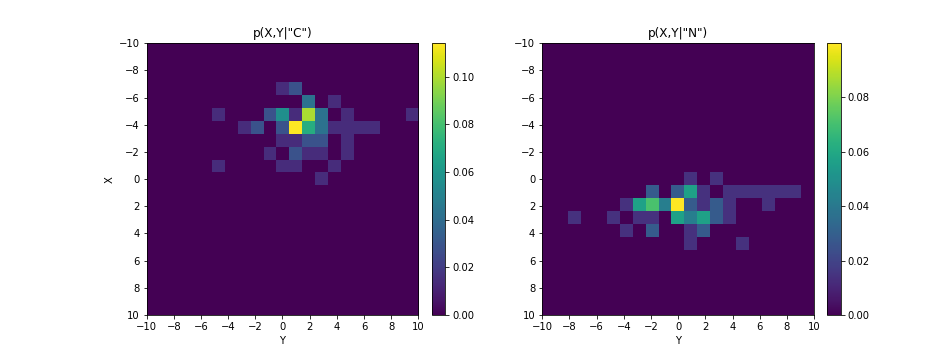1. Complete the template of the function minimax_strategy_discrete. It should return the optimal Minimax strategy, optimal index $i^*$, and $\epsilon_1$ and $\epsilon_2$ arrays. Where the LR is $0/0 = \mbox{nan}$, prefer the first class (this is needed for automatic evaluation). Also only search over indices from 0 up, $i^* \in [0, N)$, (caused by our mistake)

Hint: np.sort and np.argsort handle $\mbox{nan}$ reasonably for the search.

1. Plot $\epsilon_1$ and $\epsilon_2$ for varying index $i$ together with the found optimal index $i^*$. Use the provided function plot_lr_threshold. Save the figure to minimax_lr_search_discrete.png.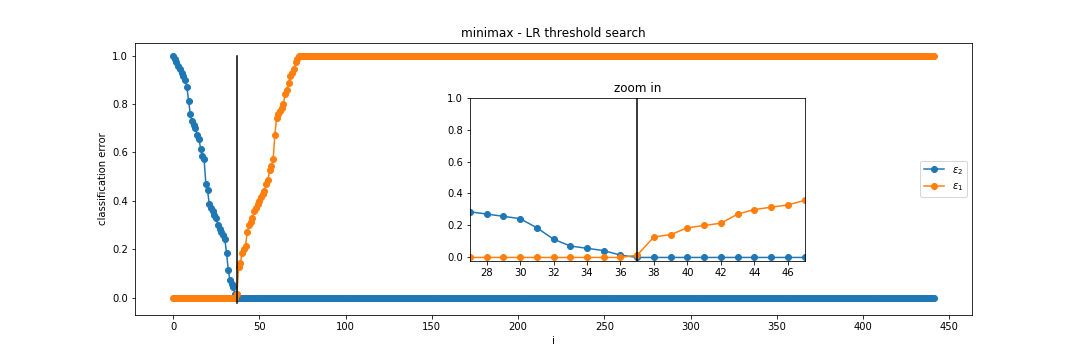1. Plot the found optimal strategy using plot_discrete_strategy and save the figure as minimax_strategy_discrete.png.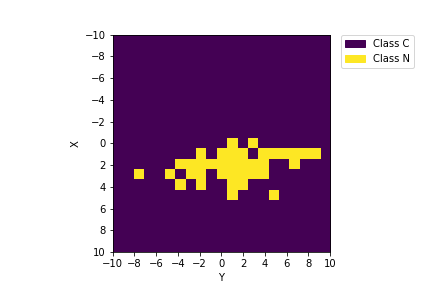1. Complete the function classify_discrete which takes a strategy and a set of image measurements (from two classes) and produces the classification labels.

1. Show the classification and save the figure as minimax_classif_discrete.png.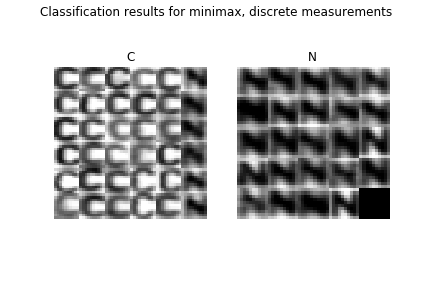## Continuous measurements

In this section we will be using a continuous measurement $x$ computed by compute_measurement_lr_cont.

The class probability density functions are assumed to be normal distributions and they are for every letter specified in the cont[letter] variable. So, for instance for the letter “A”, the mean is in cont['A']['Mean'] and the standard deviation in cont['A']['Sigma'].

Again, we will select only two letters (e.g. your initials) from the alphabet and consider only the two-class problem with labels 1 and 2.

Before finding the optimal Minimax strategy for the continuous case we will spend some time drawing various Bayes risks to demonstrate the relation of the Minimax and the Bayes problems.

Step by step, we will build the following graph and then we find the optimal Minimax strategy based on our understanding of the relation between Minimax and Bayes.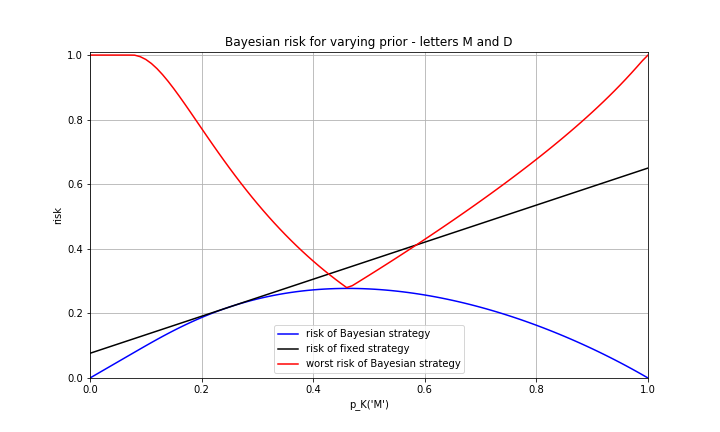1. For $p_K(1) \in \{0, 0.01, \ldots, 0.99, 1\}$ compute and plot into one figure the following:
1. Risk of the Bayesian strategy optimal for each $p_K(1)$ (the blue curve).

Hint 1: Use your code from the last labs.

Hint 2: In the Minimax problem formulation, each error is penalised equally independent of the class which corresponds to the 0-1 loss function.

2. Find the optimal strategy for $p_K(1) = 0.25$. Then for this, now fixed strategy, alter the a priori probability and compute its Bayesian risk (black line). For this complete the template function risk_fix_q_cont.

Hint 1: This is what happens, when we have assumed wrong a priori probabilities different from the real one.
Hint 2: Equation (3) from  explains the shape of the observed curve.

3. For each iterated $p_K(1)$ derive the optimal Bayesian strategy and compute its worst-case risk in case the true a priori probability is different (red curve). For this complete the template function worst_risk_cont.

Hint: You can again use the equation (3) from  and the fact that the a priori probability is bounded to the <0,1> interval.

2. Save the figure to plots_cont.png.

3. Complete the template function minmax_strategy_cont, so that it finds the Minimax strategy and its risk.

Hint: The Minimax strategy is the Bayesian strategy with minimal maximal Bayesian risk over all $p_K(C)$ (it is not enough to minimize over bunch of pre-set priors) .

Hint: use scipy.optimize.fminbound with a helper (e.g. lambda) function that has just one parameter (the prior) and returns the corresponding risk

def orig_function(w, x, y, z):
return w * x * y * z

w_val = 10
y_val = 11
z_val = 12
helper_function = lambda prior: orig_function(w_val, prior, y_val, z_val)

...results... = fminbound(helper_function, -42.0, 42.0, full_output=True)

D1 = cont['M']
D2 = cont['D']
q, risk = minmax_strategy_cont(D1, D2)

# q['t1'] -> -230.44601288127865
# q['t2'] -> 3705.0796592073866
# q['decision'] -> array([1, 0, 1], dtype=int32)
# risk -> 0.2774251103401184
4. Plot Bayesian risk for all values of $p_K(1)$ for the minimax strategy.

5. Generate a test set for your two letters using create_test_set.

6. Compute the error of the strategy on the generated test images, show the final classification in one figure and save it as minmax_classif_cont.png.

measurements_cont = compute_measurement_lr_cont(images_test_cont)
labels_estimated_cont = classify_2normal(measurements_cont, q)
error_cont = classification_error(labels_estimated_cont, labels_test_cont)

# error_cont -> 0.3167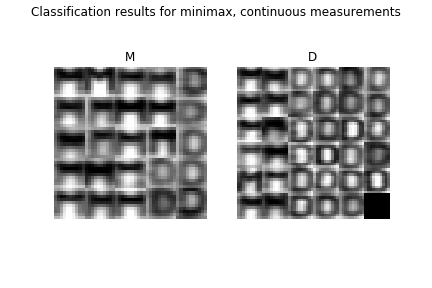## Assignment Quiz

1. What is the maximal risk of the optimal Bayesian strategy trained for $P_K(A)=0.3$ and $P_K(B)=0.7$ using continuous measurement when the a priori probabilities are allowed to change? Round it to two decimal points.

Solution

2. The relation between the worst case risk and the best case risk for the minimax strategy in the continuous case is

• a) The worst case risk is always greater than the best case risk
• b) The worst case risk could be smaller than the best case risk
• c) The worst case risk is strictly equal to the best case risk

Solution

3. In the above we assumed the a priori probability exists but is not known. What would change if it didn't exist?

• a) We can't compute the risk and have to use the likelihood ratio directly
• b) We would need to use different loss function W
• c) We would proceed the same way as we did above

Solution

Go through the chapter on non-Bayesian tasks in SH10 book , especially the parts discussing solution of the minimax task by linear programming (pages 25, 31, 35-38, 40-41, 46-47). Solve the above classification task using linear programming as described on page 47.

Hints:

• Work with the discrete measurements $x$.
• Represent the sought strategy (classification function) as a table α, with α(i,k) corresponding to the probability of classification of bin 'i' to class 'k' such that α(i,k) >= 0 and sum_k α(i,k) = 1.
• Reformulate the task in a matrix form according to equation 2.53 in SH10, page 47
• Solve the task numerically using scipy.optimize.linprog.
• Compare the obtained results with the results of the classification above.
•  Minimax Task (short support text for labs)
•  Michail I. Schlesinger, Vaclav Hlavac. Ten Lectures on Statistical and Structural Pattern Recognition. Kluwer Academic Publishers, 2002. (available in CMP library or online)